# Retrieval of long-wave tsunami Green’s function from the cross-correlation of continuous ocean waves excited by far-field random noise sources on the basis of a first-order Born approximation

## Abstract

We investigate the theoretical background for the retrieval of the tsunami Green’s function from the cross-correlation of continuous ocean waves. Considering that a tsunami is a long-wavelength ocean wave described by 2-D linear long-wave equations, and that the sea-bottom topography acts as a set of point-like scatterers, we use a first-order Born approximation in deriving the tsunami Green’s function having coda waves. The scattering pattern is non-isotropic and symmetrical with respect to the forward and backward directions. We indicate a retrieval process which shows that the derivative of the cross-correlation function of wavefields at two receivers with respect to the lag time gives the tsunami Green’s function when point noise sources generating continuous ocean waves are distributed far from, and surrounding, the two receivers. Note that this relation between the cross-correlation and the Green’s function is different from the case in which uncorrelated plane-wave incidence from all directions is assumed to be continuous ocean waves. The Green’s function retrieved from continuous ocean waves will be used as a reference to examine the validity of the Green’s function obtained by numerical simulations.

## 1. Introduction

The technique of seismic interferometry, whereby the seismic Green’s function between two points is extracted from the cross-correlation of ambient seismic noise, has received a great deal of attention from seismologists (e.g., Campillo and Paul, 2003). Using this technique, seismologists can calculate the Green’s function, or estimate subsurface structures, by analyzing ambient noise, without the need for natural or artificial earthquakes (e.g., Shapiro et al., 2005; Nishida et al., 2009). For tsunami researchers, it is necessary to use the correct tsunami Green’s functions for tsunami source inversion analysis (e.g., Satake, 1989; Saito et al., 2010) or for simulating disastrous tsunamis generated by past, and anticipated future, huge earthquakes (e.g., Furumura et al., 2011). The correct tsunami Green’s function should be obtainable by numerical simulation using accurate and high-resolution bathymetry data. However, we cannot always obtain the correct Green’s function through numerical simulations. For example, in the 2010 Maule, Chile, earthquake tsunami, there was a significant discrepancy (30 min) between the observed, and simulated, arrival times around Japan (Satake et al., 2010). Therefore, it would be very useful if we are able to synthesize the tsunami Green’s function from observed “ambient” ocean waves; in other words, if we can retrieve the tsunami Green’s function from the cross-correlation of continuous ocean waves observed by, for example, ocean-bottom pressure gauges. Therefore, we investigate the theoretical background for the retrieval of the tsunami Green’s function from the cross-correlation of continuous ocean waves. This is the first attempt to demonstrate a retrieval process of the tsunami Green’s function which, in particular, includes coda waves. Considering that the tsunami has a long wavelength and the sea-bottom topography acts as a set of pointlike scatterers, we use a first-order Born approximation. The framework of the present study follows Sato (2009), who dealt with the case of 3-D scalar waves with isotropic scattering. For the application to a tsunami, the present study extends the approach of Sato to the case of 2-D waves with special non-isotropic scattering.

## 2. Tsunami Green’s Function in a Scattering Medium: Single Scattering

We assume that ocean waves and tsunamis obey a 2-D linear long-wave equation (e.g., equation (2) in Saito and Furumura, 2009) in x-y coordinates. The sea depth is represented as, where h0 is the average sea depth, and h0ξ (x) is the fluctuation from the average. The fluctuation is assumed to be small, |ξ (x)| 1. The wave equation for an ocean wave, or tsunami, η (x, t), for a noise source N(x, t), is then given as follows: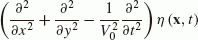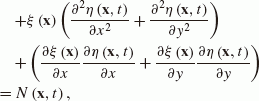(1)

where, and g0 is the gravitational acceleration. We solve Eq. (1) for a delta-function source term in the frequency domain, in order to obtain the Green’s function. The Green’s function Ĝ (x, x0, ω) for a source located at x = x0 satisfies the following equation:(2)

where the wavenumber k0 is given by k0 = ω/V0. The Green’s function Ĝ0 (x, x0, ω), for the constant sea depth h = h0, satisfies the following equation:(3)

and is given by,(4)

whereis the first Hankel function of order zero (e.g., Snieder, 2001). We assume that a receiver is located at x A = (h, 0), and that a source is located at x B = (−h, 0), on the x-axis, with a separation distance of r AB = 2h (Fig. 1). Based on Sato (2009), the sea-bottom fluctuation ξ (x) is assumed to be represented as, where ε j is a scale factor adjusting the sea-bottom topography fluctuation and the spatial scale d is small compared with the tsunami wavelength. The sea-bottom fluctuation ξ (x) then acts as a set of point-like scatterers. The scatterers are located within a region of size D around x A and x B . The far-field scattered wave from the scatterers is theoretically calculated by using a first-order Born approximation. We then obtain the Green’s function for Eq. (2) (Saito and Furumura, 2009) as follows: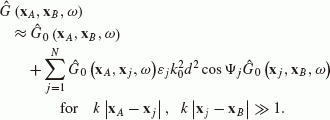(5)

The angle Ψ j is the scattering angle for the j th scatterer located at y j and satisfies,(6)

where θ Aj (or θ Bj ) is the angle between the x-axis and the line connecting x A (or x B ) and y j (Fig. 2). The second term in Eq. (5) represents coda waves composed of single scattering waves, which is similar to Eq. (3) of Sato (2009). However, unlike the isotropic scattering for the scalar wave in Sato (2009), this term indicates non-isotropic scattering as characterized by Eq. (6) for a long-wave tsunami.

## 3. Cross-correlation Function of Waves in a Scattering Medium Illuminated by Surrounding Noise Sources

Noise sources with a spectrumare distributed on a circle of radius R with a small width ΔR, where R is much greater than D (Fig. 1). The continuous ocean wave at location x A , excited by the noise sources, is given in terms of polar coordinates (r, θ) by,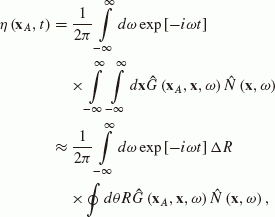(7)

where θ is measured from the x-axis, and the symbol to θ over a range of 2π. We imagine an ensemble of noise sources. When the locations of sources are not correlated, we may write the ensemble average of the noise spectra as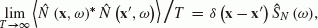, where the * symbol represents a complex conjugate, T is the time window length, and Ŝ N (ω) is the power spectral density function of stationary noise (Sato, 2009). Note thatbecause N (x, t) is real. We define the cross-correlation function of continuous ocean waves observed at x A and x B over the ensemble of noise sources as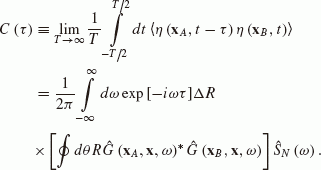(8)

Substituting Eqs. (4) and (5) into Eq. (8), we calculate the integral with respect to θ. In this calculation, we use the approximation in the denominatorsas geometrical spreading factors, because the noise sources are distributed in the far field, R D. We also approximate the distance in the exponent, and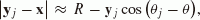, where. These approximations are common to Sato (2009). The scattering angles Ψ Aj and Ψ Bj are given by θ Aj θ and θθ Bj , respectively (Fig. 3). We then obtain the following equation for the integration with respect to θ in Eq. (8):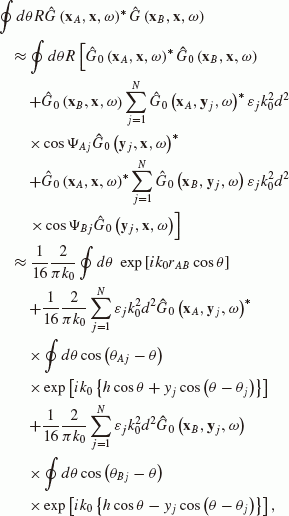(9)

where the second-order terms with respect to ε j are neglected. For the calculation of the integral in the second term of Eq. (9), we use the relations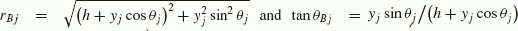as shown in Fig. 4, and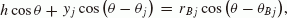, where r Bj (or r Aj ) is the distance between x B (or x A ) and y j . We then obtain,(10)

where J1 is a Bessel function of the first order. Similarly, with the relation, the third term of Eq. (9) is also calculated. Then, using Eq. (4), we rewrite Eq. (9) as follows:(11)

where J0 and N0 are Bessel and Neumann functions of order zero, respectively. Assuming a far field k0r 1 and using the approximation,(Abramowits and Stegun, 1972), we finally obtain,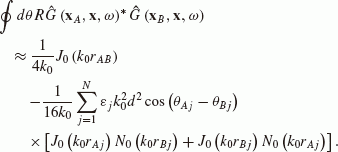(12)

Now, in order to compare Eq. (12) with the imaginary part of Eq. (5), we rewrite Eq. (5), by using Eq. (4), as follows:(13)

Finally, comparing Eqs. (12) and (13), we obtain the following relation:(14)

for the 2-D tsunami wave equation including the scattered waves from the point-like scatterers. This form of the 2-D tsunami equation is consistent with the counterpart for the 3-D case (e.g., equation (13) in Sato (2009)). Based on Eqs. (8) and (14), we obtain the relation in the time domain as follows: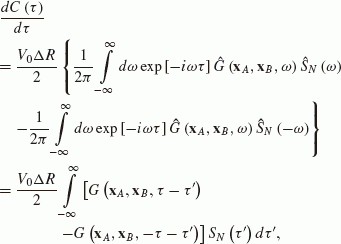(15)

where G (x A , x B , t) is the Green’s function in the time domain and S N (τ) is the auto-correlation function of the noise sources. In the calculation, we used Ĝ (x A , x B , ω)* = Ĝ (x A , x B , −ω), because G (x A , x B , t) is real, and Ŝ N (−ω) = Ŝ N (ω) because S N (−τ) = S N (τ).

In particular, for the noise spectrum, which is assumed to be white, given by Ŝ N (ω) = S0 and S N (τ) = S0δ (τ), Eq. (15) becomes,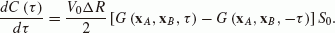(16)

Equation (16) indicates the relation between the spatial correlation of continuous ocean waves and the tsunami Green’s function. The derivative of the cross-correlation function for the two receivers with respect to lag time gives the Green’s function.

Equation (16) for the 2-D tsunami is consistent with the counterpart for the 3-D scalar wave (e.g. Roux et al., 2005; Sato, 2009). Note, however, that this form is different from that derived by Nakahara (2006) for 2-D scalar waves, in which the Hilbert transform of the cross-correlation function gives the Green’s function. The difference comes from the assumption of the noise sources. The present study assumes point noise sources, whereas Nakahara (2006) assumes uncorrelated plane-wave incidence as a noise wave-field. In 2-D space, a cylindrical wave impulsively radiated from a point source exhibits waveform broadening with a lapse time even in homogeneous media. By contrast, a plane wave maintains its initial shape during the propagation. This causes the difference between the results obtained in the present study and those of Nakahara (2006), in which the broadening nature of the Green’s function is recovered by Hilbert-transforming the cross-correlation function.

## 4. Numerical Examples

In order to validate the theoretical results of the present study, we performed numerical simulations based on the boundary integral method of Yomogida and Benites (1995), which was originally developed for simulating SH wave scattering in 2-D elastic media with cavities. We herein apply this method to tsunami scattering. This is justified by the fact that the 2-D wave equation of a tsunami (Eq. (1)), and that of SH waves in an elastic media of constant density are mathematically equivalent. In this relationship between the tsunami and the SH waves, a cavity in the elastic media corresponds to an island (a small area with zero sea depth).

We take two receivers with a separation distance of 100 km, and one circular island with a radius of 10 km as a point-like scatterer, which are surrounded by 3, 000 noise sources (Fig. 5(a)). Here, V0 = 0.1 km/s, R = 300 km, and ΔR = 100 km, and the distance between the scatterer and either receiver is 112 km. Each noise source radiates a Ricker wavelet with a dominant frequency of 0.003 Hz and a corresponding wavelength of 33 km, which is larger than the size of the scatterer. We numerically simulated the wave propagation from the noise sources and synthesized the wavefields at the two receivers. Figure 5(b) shows the time derivative of the cross-correlation function of these wavefields (corresponding to the left-hand side of Eq. (15)). We also synthesized the Green’s function between the two receivers (convolved with the auto-correlation function of the noise sources corresponding to the right-hand side of Eq. (15)), which is also shown in the figure. The agreement between the waveforms is confirmed to be excellent.

As a more realistic example, we placed land (half space with zero sea depth) beside the receivers (Fig. 5(c)). The distance between the land s coast and the nearest receiver is 150 km. Although the number of noise sources is unchanged, there are no noise sources in the land. The tsunami reflected from the coast is recognized at ±4, 000 s in Fig. 5(d). Compared with the case of Fig. 5(b), the waveform retrieved from the cross-correlation function partly disagrees with the synthesized Green’s function. In particular, the amplitude with respect to the time axis is significantly asymmetric in the Green’s function retrieved from the cross-correlation function, because of the uneven distribution of the noise sources in space. Nevertheless, the arrival times of the maximum-amplitude wave, the scattered wave, and the wave reflected from the land in the Green’s function retrieved from the cross-correlation function explain well these arrival times for the synthesized Green function. This indicates that the retrieved Green’s function can be used as a reference in examining the validity of the travel time as calculated by numerical tsunami simulations.

## 5. Concluding Remarks

The present paper describes a retrieval process for the 2-D tsunami Green’s function from the cross-correlation of continuous ocean waves based on a first-order Born approximation. Equation (16) indicates that the derivative of the cross-correlation function for two receivers with respect to lag time gives the tsunami Green’s function including coda waves. Furthermore, we have presented numerical examples of the Green’s function retrieved from the cross-correlation function of the continuous waves to confirm the validity of the obtained results.

We herein assumed single scattering for the coda excitation. On the other hand, Margerin and Sato (2011) demonstrated the effects of a multiple scattering process in the retrieval of the 3-D scalar-wave Green’s function using Feynman diagrams. Some studies have also taken the higher-order perturbation of waves into consideration for the retrieval process (Sanchez-Sesma et al., 2006; Snieder et al., 2008; Wapenaar et al., 2010). A general theory of Green’s function retrieval for linear partial differential equations was recently presented by Fleury et al. (2010). Such general theories, which are not (or, at least, not strongly) restricted by the approximation conditions, are obviously important. On the other hand, the simplicity of analyzing specific situations, such as tsunami propagation with single scattering, as considered in the present study, may also be useful. For example, such an analysis would be helpful in interpreting the numerical simulation results, or practical analyses, of observed tsunami records. For the case of tsunami propagation, since accurate high-resolution bathymetric data is available, it would be interesting to simulate the retrieval of the tsunami Green’s function using actual bathymetry data. The results reported herein will be helpful in interpreting the features of actual records and the retrieved Green’s functions.

## References

• Abramowits, M. and I. A. Stegun, Handbook of Mathematical Functions, Dover, Mineola, N.Y., 1972.

• Campillo, M. and A. Paul, Long range correlations in the seismic coda, Science, 299, 547–549, 2003.

• Fleury, C., R. Snieder, and K. Larner, General representation theorem for perturbed media and application to Green’s function retrieval for scattering problems, Geophys. J. Int., 183, 1648–1662, doi:10.1111/j.1365-246X.2010.04825.x, 2010.

• Furumura, T., K. Imai, and T. Maeda, A revised tsunami source model for the 1707 Hoei earthquake and simulation of tsunami inundation of Ryujin Lake, Kyushu, Japan, J. Geophys. Res., doi:10.1029/2010JB007918, 2011.

• Margerin, L. and H. Sato, Reconstruction of multiply-scattered arrivals from the cross-correlation of waves excited by random noise sources in a heterogeneous dissipative medium, Wave Motion, doi:10.1016/j.wavemoti.2010.10.001, 2011.

• Nakahara, H., A systematic study of theoretical relations between spatial correlation and Green’s function in one-, two- and three-dimensional random scalar wavefields, Geophys. J. Int., 167, 1097–1105, doi:10.1111/j.1365-246X.2006.03170.x, 2006.

• Nishida, K., J. P. Montagner, and H. Kawakatsu, Global surface wave tomographyusing seismic hum, Science, 326(5949), 112, 2009.

• Roux, P., K. G. Sabra, W. A. Kuperman, and A. Roux, Ambient noise cross correlation in free space: Theoretical approach, J. Acoust. Soc. Am., 117, 79–84, 2005.

• Saito, T. and T. Furumura, Scattering of linear long-wave tsunamis due to randomly fluctuating sea-bottom topography: Coda excitation and scattering attenuation, Geophys. J. Int., 177, 958–965, doi:10.1111/j.1365-246X.2009.03988.x, 2009.

• Saito, T., K. Satake, and T. Furumura, Tsunami waveform inversion including dispersive waves: The 2004 earthquake off Kii Peninsula, Japan, J. Geophys. Res., 115, B06303, doi:10.1029/2009JB006884, 2010.

• Sanchez-Sesma, F. J., J. A. Perez-Ruiz, M. Campillo, and F. Luzon, Elastodynamic 2D Green function retrieval from cross-correlation: Canonical inclusion problem, Geophys. Res. Lett., 33, L13305, doi:10.1029/2006GL026454, 2006.

• Satake, K., Inversion of tsunami waveforms for the estimation of heterogeneous fault motion of large submarine earthquakes: The 1968 Tokachioki and 1983 Japan Sea earthquakes, J. Geophys, Res., 94, 5627–5636, doi:10.1029/JB094iB05p05627, 1989.

• Satake, K., S. Sakai, T. Kanazawa, Y. Fujii, T. Saito, and T. Ozaki, The February 2010 Chilean tsunami recorded on bottom pressure gauges, MIS050-P05, Japan Geoscience Union Meeting 2010, 2010.

• Sato, H., Retrieval of Green’s function having coda from the cross-correlation function in a scattering medium illuminated by surrounding noise sources on the basis of the first order Born approximation, Geophys. J. Int., 179, 408–412, doi:10.1111/j.1365-246X.2009.04296.x, 2009.

• Shapiro, N. M., M. Campillo, L. Stehly, and M. Ritzwoller, Highresolution surface wave tomography from ambient seismic noise, Science, 307, 1615–1618, 2005.

• Snieder, R., A Guided Tour of Mathematical Methods for the Physical Sciences, Cambridge Univ. Press, Cambridge, U.K., 2001.

• Snieder, R., K. van Wijk, M. Haney, and R. Calvert, Cancellation of spurious arrivals in Green’s function extraction and the generalized optical theorem, Phys. Rev. E, 78, 036606, 2008.

• Wapenaar, K., E. Slob, and R. Snieder, On seismic interferometry, the generalized optical theorem, and the scattering matrix of a point scatterer, Geophysics, 75, SA27–SA35, doi:10.1190/1.3374359, 2010.

• Yomogida, K. and R. A. Benites, Relation between direct wave Q and coda Q: A numerical approach, Geophys. J. Int., 123, 471–483, 1995.

## Acknowledgements

The authors would like to thank K. Yomogida for allowing the use of his computer code for the boundary integral simulations. We would also like to thank H. Sato and H. Nakahara for their useful discussions and advice. The manuscript was greatly improved by the careful reading and comments provided by T. Hara and the two anonymous reviewers.

## Author information

Authors

### Corresponding author

Correspondence to Tatsuhiko Saito.

## Rights and permissions

The images or other third party material in this article are included in the article’s Creative Commons licence, unless indicated otherwise in a credit line to the material. If material is not included in the article’s Creative Commons licence and your intended use is not permitted by statutory regulation or exceeds the permitted use, you will need to obtain permission directly from the copyright holder.

To view a copy of this licence, visit https://creativecommons.org/licenses/by/4.0/.

Reprints and Permissions

Saito, T., Kawahara, J. Retrieval of long-wave tsunami Green’s function from the cross-correlation of continuous ocean waves excited by far-field random noise sources on the basis of a first-order Born approximation. Earth Planet Sp 64, 43–48 (2012). https://doi.org/10.5047/eps.2011.08.020

• Revised:

• Accepted:

• Published:

• Issue Date:

• DOI: https://doi.org/10.5047/eps.2011.08.020

### Key words

• Tsunami
• theory
• interferometry